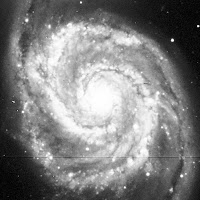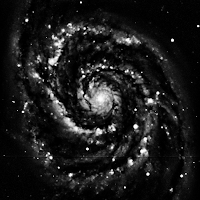## Introduction

Here we take a look at the optimisation of cool image processing algorithm, John Gauch's Weighted Region Ranking, using OpenMP. Basically, wrr is an adaptive contrast enhancement algorithm which maximises the contrast locally, for each pixels, in relation to their neighbours, up to a defined extent.

John Gauch puts it better than I could:
There are a number of non-linear methods for enhancing the details in images. One approach I have investigated is a variation of ahe called weighted region ranking. Here a circular neighborhood about each point is searched to count the number of points lighter and darker than the central point. This gives us the rank of each pixel which we display as an output image. The size of the search region and the amount of histogram smoothing prior to ranking control the amount of enhancement performed.
I used SWIG and numpy.i to wrap the code for Python. The single threaded code and multi-threaded were tested using the same simple testing script.

The 512x512 16 bit image I chose for testing is a very nice picture of the M51 galaxy in the astronomical FITS format, as downloaded from this SAO Telescope Data Center page (original source: Kitt Peak National Observatory).

## FITS dataM51, histogram equalized image
My purpose was to get a nice 16 bit image to work with, not to delve into the FITS format. In any case, PyFITS was most useful, and I've included a little python script to convert FITS to 16 bit compressed TIFF (using pylibtiff) and to also save an 8 bit equalized image in both PNG and JPEG.

## Reformating wrr.c for wrapping with SWIG/numpy.i

I didn't change much in the original wrr.c file, just the function prototype and one helper function to create the table of line pointers. This is what I really like about working with SWIG: The Python logic (contained in wrr.i) is kept separate from the actual code (wrr.c).

### Modified wrr function prototype:

`void wrr(int *ImageIn, int Ydim, int Xdim, int nbits, float BlurStdDev, float WeightStdDev, int Radius, int Step, int **ImageOut, int *YdimOut, int *XdimOut);`
• int *ImageIn, int Ydim, int Xdim will be the 32 bit numpy array data coming from Python
• nbits (subject to change) is the number of bits of useful information in the 32 bit output array (so up to 31 as I used signed integer arrays)
• BlurStdDev, WeightStdDev, Radius as defined on the wrr page
• Step indicates the step size over the region of interest for calculating the pixel rank. For a 40x40 neighbourhood for example, a step size of 4 means only 100 equidistant pixels are considered instead of the 40x40 = 1600 pixels. This may introduce some artifacts, so use with caution.
• int **ImageOut, int *YdimOut, int *XdimOut is wrapped by SWIG into a memory managed numpy array. The memory allocated for the array will be automatically de-allocated once the numpy array is destroyed, as outlined here.
Just as a historic side note, when I first experimented with the algorithm back in 1995, the machine I needed to run the algorithm on was a 486 DX2-66. I used a stripped down version of the Region Ranking algorithm which I compiled in DPMI mode using DJGPP. Even with the stepping trick, processing a 640x640 digital Chest X-Ray image still took 15 seconds.

### Line pointer table creation:

Creating these tables greatly improves the readability of C code. Instead of array[y*width+x], one can write line_array[y][x]. The following code is used to create the line_array table (myalloc.c):

```void **make_strides(void *Image, int Ydim, int Xdim, size_t size)
{
char *ptr;
void **out = NULL;
size_t stride;
int i;

if (Image == NULL)
{
errno = ENOMEM;
goto end;
}

//assumes pointers are all the same size.
out = (void **)malloc(Ydim*sizeof(void *));
if (out == NULL)
{
errno = ENOMEM;
goto end;
}

//stride is image line size bytes
stride = size * Xdim;

//we're using a char pointer for 1 byte increments.
ptr = (char *)Image;

for (i=0; i<Ydim; i++)
{
out[i] = (void *)ptr;
//now can increment ptr in bytes using stride
ptr+=stride;
}

end:
return out;
}
```

Hopefully the code is simple enough to understand. If I missed something, don't hesitate to point it out!

A typical call to make_strides would therefore look like:

```    /* Allocate weight array */
weight_size = (2 * Radius + 1);
ImageWeight = (float *)malloc(weight_size * weight_size * sizeof(float));
Weight = (float **)make_strides(ImageWeight, weight_size, weight_size, sizeof(float));
```

OK, time to check what wrr does on our M51 image.

The BlurStdDev value comes-in handy for removing the background noise. You will want that pretty high, although there's a definite computational cost associated with it.

Here we check increasing values of Radius (5,10,20,40).
Other parameters: BlurStdDev = 100.0, WeightStdDev = 20.0, Step = 1Radius=5 Radius=10Radius=20 Radius=40
Processing time increases with Radius. A simple test conducted on an Intel Core2 Quad Q8300 @ 2.50GHz, gcc version 4.6.3 (Ubuntu/Linaro 4.6.3-1ubuntu5), Ubuntu 12.04 LTS, 64 bit produces the following. YMMV:

From the OpenMP webpage:
The OpenMP API supports multi-platform shared-memory parallel programming in C/C++ and Fortran. The OpenMP API defines a portable, scalable model with a simple and flexible interface for developing parallel applications on platforms from the desktop to the supercomputer.
I'm by all means no expert on OpenMP, so take what I say with a fistfull of salt. This is what I've had to do to integrate PRAGMA based OpenMP statements in the wrr function:
• I added an include <omp.h> statement to wrr.c
• I added "-fopenmp" to the compile_args list, and "-lgomp" to the extra_link_args list in setup.py
• We have 4 nested loops in wrr, y,x,dy,dx. I found that multi-threading the outer loop gave the fastest code. As far as I understand, each line in the output image is produced by a different thread.
To expand on the last point, this is the OpenMP statement I used:

```    if (BlurStdDev <= 1)
{
#pragma omp parallel for        \
default(shared) private(x,dy,dx,Area,Below,Center,Temp)

for (y = 0; y < Ydim; y++)
for (x = 0; x < Xdim; x++)
```

The most difficult part (at least at the very basic level I'm using OpenMP at) is to identify within one thread, which variables are shared and which variables are private to the thread. In this case, the loop index and temporary variables are private. Everything else, the y outer loop index, data and line pointer tables are shared.

In the second test, we need to add Blur as one of the private variables:

```    /* Handle slower case with histogram blurring */
else
{
#pragma omp parallel for        \
default(shared) private(x,dy,dx,Area,Below,Center,Temp, Blur)

for (y = 0; y < Ydim; y++)
for (x = 0; x < Xdim; x++)
{
```

... and that's it! Time to check the multi-threaded versions:
Speed-up achieved for Radius=40: The multi-threaded version (on 4 cores) is 2.6x faster than the single threaded one. There's probably room for improvement, but it's a start!

### EDIT

To get a better idea of the code speed-up (wall time) and thread creation impact (CPU clock time), I wrote a small test case in C (use the Makefile).

Depending on what's running in the background, output will look something like this:

./test_wrr -n 5

iteration 1/5, 0.794s
iteration 2/5, 0.792s
iteration 3/5, 0.791s
iteration 4/5, 0.791s
iteration 5/5, 0.791s
mean for 5 repeats: 0.792s

iteration 1/5, 0.242s
iteration 2/5, 0.238s
iteration 3/5, 0.243s
iteration 4/5, 0.244s
iteration 5/5, 0.240s
mean for 5 repeats: 0.241s